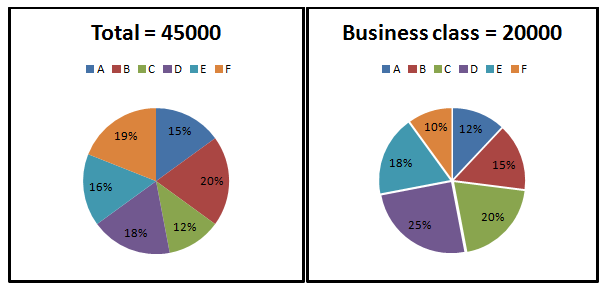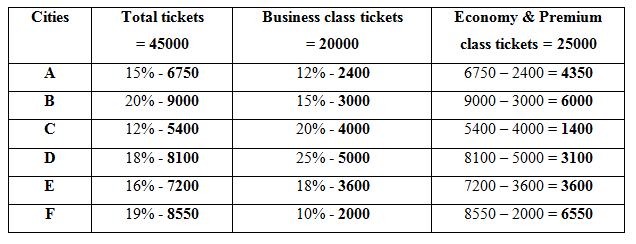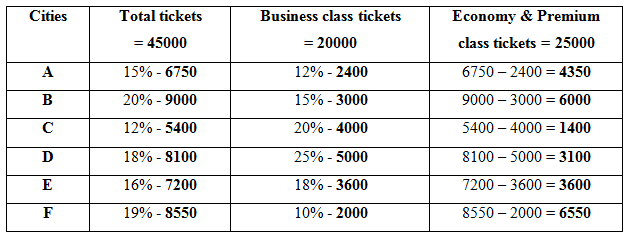# IBPS Clerk Mains Quantitative Aptitude Questions 2019 (Day-12) High Level New Pattern

Dear Aspirants, Quantitative Aptitude plays a crucial role in Banking and all other competitive exams. To enrich your preparation, here we have provided New Pattern Aptitude Questions for IBPS Clerk Mains. Candidates those who are going to appear in IBPS Clerk Mains can practice these questions daily and make your preparation effective.

[WpProQuiz 4766]

Directions (1-5): Read the following information carefully and answer the given questions.

The pie chart 1 shows the percentage of total number of tickets booked in different cities from three different classes (Business class, Economy class and Premium class).

The pie chart 2 shows the percentage of total number of business class tickets booked in different cities.1) Quantity I: Find the Premium class tickets booked in the city which is the highest number of tickets booked other than the business class in the given cities, if the ratio of Economy class and Premium class is 70: 61

Quantity II: Find the highest number of business class tickets booked in the given cities

a) Quantity I > Quantity II

b) Quantity I < Quantity II

c) Quantity I ≥ Quantity II

d) Quantity I ≤ Quantity II

e) Quantity I = Quantity II (or) Relationship cannot be determined

2) Find the difference between the total business class tickets booked in the cities of D and F together and the total tickets booked other than the business class in the same cities together?

a) 2450

b) 2440

c) 2650

d) 2560

e) 2540

3) What is the ratio of the number of males to females booked the business class tickets in the cities B and F together?

Statement I: If the ratio of the number of females booked business class tickets in the cities B to that of F is 5: 3

Statement II: If the ratio of the number of males booked business class tickets in the cities B to that of F is 5: 4

a) Only I

b) Only II

c) Either I or II

d) Both are not sufficient

e) Both are sufficient

4) Total number of tickets booked other than the business class in the cities A and B together is approximately what percentage of the total number of tickets booked business class in the cities C and E together?

a) 152%

b) 124%

c) 104%

d) 136%

e) 183%

5) What is the ratio of the total number of premium class tickets booked in the cities D and F together to that of the total number of economy class tickets booked in the same cities?

Statement I: Number of premium class tickets booked in the city D is 2050 less than the number of premium class tickets booked in the city F.

Statement II: Number of economy class tickets booked in the city F is 1400 more than the number of economy class tickets booked in the city D.

Statement III: Number of premium class tickets booked in the city F is 450 less than the number of Economy class tickets booked in the same city.

a) Any of the two statements

b) Only II and III together

c) Only I and III together

d) Both options (b) and (c)

e) All the statements are not sufficient

Directions (6 – 8): Read the following information carefully and answer the given questions.

Abhi, Bhavan and Charlie started a business and invested Rs. 40000, Rs. 60000 and Rs. 70000 respectively. At the end of first quarter, they invested additional amount in the ratio of 1: 2: 1. At the end of second quarter, they invested additional amount in the ratio of 4: 1: 10. At the end of third quarter, they invested additional amount in the ratio of 3: 2: 3.

6) If Abhi and Bhavan’s profit ratio at the end of second quarter is 9: 14, then find the additional amount invested by Charlie at the end of first quarter?

a) Rs.15000

b) Rs.12000

c) Rs.10000

d) Rs.16000

e) Rs.18000

7) If the ratio of additional amount invested by Abhi at the end of first quarter, second quarter and third quarter is 5: 2: 3, then find the ratio of the additional amount invested by Charlie?

a) 5: 3: 5

b) 3: 5: 5

c) 4: 3: 5

d) 5: 5: 3

e) Cannot be determined

8) Find the profit ratio of Abhi, Bhavan and Charlie at the end of third quarter?

Statement I: If the additional amount invested by Charlie at the end of first quarter and second quarter equally.

Statement II: If the additional amount invested by Bhavan at the end of first quarter is Rs.1000 more than the second quarter.

a) Both are sufficient

b) Both are not sufficient

c) Either I or II

d) Only II

e) Only I

9) Ravi invested Rs._____ and Rs._____ in two schemes A and B respectively. In scheme A offers 10% compound interest compounded annually for two years. In scheme B offers 20% simple interest for 3 years. Interest received in scheme A is Rs. 900 less than the interest received in scheme B

Which of the following satisfies the two blanks given in the questions?

1. Rs.10000, Rs.5000
2. Rs.12000, Rs. 7500

III. Rs.15000, Rs.8000

a) Only I

b) Only II

c) Only III

d) Both options a) and c)

e) Both options b) and c)

10) Biman alone can complete a piece of work in 25 days. With the help of Sohan, he can complete the work in __________ days. Sohan and Milan together can complete the work in 10 days. Milan alone can complete the work in _______________ days.

Which of the following satisfies the two blanks given in the question?

a) 157/11, 15

b) 10, 30

c) 100/9, 20

d) 12, 25

e) None of these

Directions (1-5):Quantity I: Find the Premium class tickets booked in the city which is the highest number of tickets booked other than the business class in the given cities, if the ratio of Economy class and Premium class is 70: 61

From the above table, city F highest number of tickets booked other than the business class

Required total = 6550 *(61/131) = 3050

Quantity II: Find the highest number of business class tickets booked in the given cities.

Required total = 5000 = City D

Quantity I < Quantity II

Required difference = (3100+6550) – (5000+2000)

= 9650 – 7000 = 2650

Business class tickets booked in the city B = (15/100) *20000

= 3000

Business class tickets booked in the city F = (10/100) * 20000

= 2000

From statement I,

The ratio of the number of females booked business class tickets in the cities B to that of F = 5x: 3x

From statement II,

The ratio of the number of males booked business class tickets in the cities C to that of F = 5y: 4y

From statement I and II,

In city C,

5y + 5x = 3000 —- (1)

In city F,

4y + 3x = 2000 —– (2)

Equations (1) *4 – (2) *5

(20y + 20x) – (20y +15x) = 12000 – 10000

= > 5x = 2000

= > x = 400

Both the equations together are necessary to answer the given question.

Total number of tickets booked other than the business class in the cities A and B together

= (4350+6000) = 10350

Total number of tickets booked business class in the cities C and E together

= (4000+3600) = 7600

Required percentage = (10350/7600) * 100 = 136%Total number of Premium class & Economy class tickets booked in the city D

= 3100

Total number of Premium class & Economy class tickets booked in the city F

= 6550

Let us take number of premium class tickets booked in the city D and F are x and y respectively.

Number of Economy class tickets booked in the city D and F are (3100-x) and (6550 – y) respectively.

From statement I,

Number of premium class tickets booked in the city D is 2050 less than the number of premium class tickets booked in the city F.

X = y – 2050

X – y = 2050 —- (1)

From statement II,

Number of economy class tickets booked in the city F is 1400 more than the number of economy class tickets booked in the city D.

(6550 – y) = (3100 – x) + 1400

6550 – y = 3100 – x + 1400

2050 = y – x —- (2)

From statement III,

Number of premium class tickets booked in the city F is 450 less than the number of Economy class tickets booked in the same city.

Y = (6550 – y) – 450

= > 2y = 6100

= > y = 3050

Number of Economy class tickets booked in the city F = 3050 + 450

= 3500

From I and III together,

Number of Premium class tickets booked in the city F = 3050

Number of Premium class tickets booked in the city D = 3050 – 2050 = 1000

Number of Economy class tickets booked in the city D = 3100 – 1000 = 2100

Number of Economy class tickets booked in the city F = 3500

Required ratio = (3050+1000) : (2100+3500)

= 4050: 5600

= 81: 112

From II and III together,

Number of Economy class tickets booked in the city F = 3500

Number of Economy class tickets booked in the city D = 3500 – 1400

= 2100

Number of Premium class tickets booked in the city F = 3050

Number of Premium class tickets booked in the city D = 3100 – 2100

= 1000

Required ratio = (3050 + 1000): (2100 + 3500)

= 4050 : 5600

= 81 : 112

Hence, Statement I and III together (or) statement II and III together are sufficient to answer the given question.

Directions (6 – 8):

Let us take the additional amount invested by Abhi, Bhavan and Charlie at the end of first quarter be x, 2x, and x respectively.

Profit ratio of Abhi and Bhavan at the end of second quarter

= > (40000*3 + (40000+x)*3): (60000 *3 + (60000+ 2x)*3) = 9/14

(120000 +120000+3x) / (180000+180000+6x) = 9/14

(240000+3x) / (360000+6x) = 9/14

(240000*14+3x*14) = (9*360000+9*6x)

3360000 + 42x = 3240000 + 54x

120000 = 54x – 42x

120000 = 12x

= > x = 10000

Additional amount invested by Charlie at the end of first quarter = Rs. 10000

At the end of first quarter, they invested additional amount is x, 2x and x respectively

At the end of second quarter, they invested additional amount is 4y, y and 10y respectively

At the end of third quarter, they invested additional amount is 3z, 2z and 3z respectively

The additional amount invested by Abhi at the end of first quarter, second quarter and third quarter is 5a, 2a and 3a respectively

5a = x

Charlie invested additional amount at the end of first quarter (x) = 5a

2a = 4y

a = 2y

y = a/2

Charlie invested additional amount at the end of second quarter (10y)

= 10 *(a/2)

= 5a

3a = 3z

= > a = z

Charlie invested additional amount at the end of third quarter (3z) = 3a

Required ratio = 5a: 5a: 3a = 5: 5: 3

At the end of first quarter, they invested additional amount is x, 2x and x respectively

At the end of second quarter, they invested additional amount is 4y, y and 10y respectively

From statement I,

If the additional amount invested by Charlie at the end of first quarter and second quarter equally

X = 10y —- (1)

From statement II,

If the additional amount invested by Bhavan at the end of first quarter is Rs.1000 more than the second quarter

2x – y = 1000 —- (2)

Substitute equation (1) in equation (2), we get

20y – y = 1000

19y = 1000

Y = 1000/19

X = 10*(1000/19) = 10000/19

Hence, statement I and II together are sufficient to answer the given question.

Compound interest for two years shortcut,

= > x + x + x2/100

= > 10 + 10 + 100/100

= > 21%

Let us take sum invested in scheme A and B are x and y respectively

Interest received in scheme A = (21/100) * x

= 21x/100

Interest received in scheme B = 3*(20/100)*y

= 60y/100

60y/100 – 21x/100 = 900

The options should satisfy the above condition.

From I,

5000 *(60/100) – (21/100) * 10000 = 900

3000 – 2100 =900

900 = 900

This option satisfies the given condition.

From II,

12000 * (60/100) – 7500 * (21/100) = 900

7200 – 1575 = 900

5625 = 900

This does not satisfy the given condition.

From III,

15000 *(60/100) – 8000 *(21/100) = 900

9000 – 1680 = 900

7320 = 900

This does not satisfy the given condition.

Option (a)

1/Milan = 1/15

1/Sohan + 1/Milan = 1/10

=> 1/Sohan + 1/15 = 1/10

=> 1/Sohan = 1/10 – 1/15

=> 1/Sohan = (3 – 2)/30

=> 1/Sohan = 1/30

Let, Biman and Sohan can complete the work in n days.

n/25 + n/30 = 1

=> n x (6 + 5)/150 = 1

=> n = 150/11 days

This does not satisfy the given condition.

Option (b)

1/Milan = 1/30

1/Sohan + 1/Milan = 1/10

=> 1/Sohan + 1/30 = 1/10

=> 1/Sohan = 1/10 – 1/30

=> 1/Sohan = (3 – 1)/30

=> 1/Sohan = 2/30

=> 1/Sohan = 1/15

Let, Biman and Sohan can complete the work in n days.

n/25 + n/15 = 1

=> n x (3 + 5)/75 = 1

=> n = 75/8 days

This does not satisfy the given condition.

Option (c)

1/Milan = 1/20

1/Sohan + 1/Milan = 1/10

=> 1/Sohan + 1/20 = 1/10

=> 1/Sohan = 1/10 – 1/20

=> 1/Sohan = (2 – 1)/20

=> 1/Sohan = 1/20

Let, Biman and Sohan can complete the work in n days.

n/25 + n/20 = 1

=> n x (4 + 5)/100 = 1

=> n = 100/9 days

This satisfies the given condition.

Option (d)

1/Milan = 1/25

1/Sohan + 1/Milan = 1/10

=> 1/Sohan + 1/25 = 1/10

=> 1/Sohan = 1/10 – 1/25

=> 1/Sohan = (5 – 2)/50

=> 1/Sohan = 3/50

Let, Biman and Sohan can complete the work in n days.

n/25 + 3n/50 = 1

=> n x (2 + 3)/50 = 1

=> n = 50/5 = 10 days

This does not satisfy the given condition.